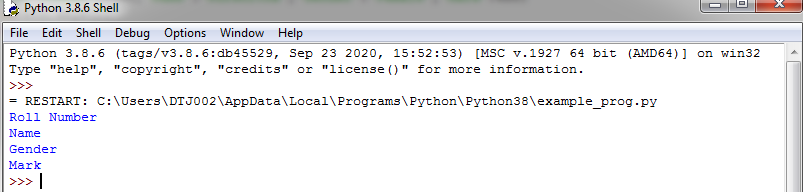## List,Tuple,Set and Dictionary in Data type models - Python

Data type models in python:

• The data type is nothing but, it tells about kinds of data.
• The data type models are used to store collection of data under a common name.

Type’s data type model:

• List
• Tuple
• Set
• Dictionary

1. List:

• The list data type is used to store the collection of an item in an ordered sequence.
• These items are mutable, which means changeable as possible.
• It is denoted by square bracket [].
• Its index value is started with 0 and ends with list_length-1.

Syntax:

1. Declaring list:

Variable_name=[]

Example:

List=[]

Declaring value into the list:

Variable_name=[item1,item2,...item n]

Example:

a=[1000,’Kurshitha’,’female’,450]

Example program:

1. a=[5,1,8,3]

print("Fist value : ",a)

print("Last value : ",a[len(a)-1])

print("Another method to print last value : ",a[-1])

print("List of elements : ",a)

Output:

Print list in looping statement:

1. a=[5,1,8,3]

for i in a:

print(i)

Output:

Insert or append an item into the list:

• To insert an element in the list is used append() function.

1. pin=[1234,1111,1211,1457]

p=int(input("Enter an item to add in list : "))

pin.append(p)

print("Your list of items are: ")

for i in pin:

print(i)

Output:

2. Tuple:

• The tuple data type is used to store collection of item in unordered and it allows duplicate values same as a list.
• These items are immutable, that means cannot be changed tuple.
• It is denoted by parentheses () but if calling the index value means using square brackets [].
• Its index value is started with 0 and end with list_length-1.

Syntax:

1. Declaring tuple:

Variable_name=()

Example:

t=()

Declaring value into the list:

Variable_name=(item1,item2,...item n)

Example:

a=(1000,’Kurshitha’,’female’,450)

Example program for print tuple elements:

1. a=(5,1,8,3)

print("Fist value : ",a)

print("Last value : ",a[len(a)-1])

print("Another method to print last value : ",a[-1])

print("List of elements : ",a)

Output:

3. Set:

• The set data type is used to store unique values and displayed by ordered sequence manner.
• These items are mutable, that means can change the sets.
• It is denoted by curly braces {} but if calling the index with value means not possible.

Syntax:

1. Declaring value into the set:

Variable_name={item1,item2,...item n}

Example:

a={1000,’Kurshitha’,’female’,450}

Example program for print the set of elements:

1. a={8,5,1,3,7}

print(a)

Output:

Insert an item into the set:

• To insert an element in the set is used add() function.

1. a={8,5,1,3,7}

print(a)

Output:

Insert number of items at a time into the set:

• To insert number of element in set is used update() function.

1. a={8,5,1,3,7}

a.update([15,10,11])

print(a)

Output:

4. Dictionary:

• The dictionary data type is similar to the sets but a little bit different and the dictionary are also mutable.
• It contains pair of parameters. i.e, keys and values.
• Key is used to store element names.
• Value is used to store element values.

Syntax:

1. Declaring dictionry:

Variable_name={}

Example:

dict={}

Declaring value into the list:

Variable_name={item_name 1: item_value 1,

item_name 2: item_value 2,

item_name n: item_value n}

Example:

std={"Roll_num":1000,'Name':'Kurshitha','Gender':'Female','mark':450}

Example program for print the dictionay of elements:

1. std={"Roll_num":1000, 'Name':'Kurshitha','Gender':'Female','mark':450}

print("Student name: ",std['Name'])

print("Elements in dictionary:")

print(std)

Output:

Print element name in for loop using keys() function:

1. std={"Roll Number":1000,'Name':'Kurshitha','Gender':'Female','Mark':450}

for i in std.keys():

print(i)

Output:Print element values in for loop using values() function:

1. std={"Roll Number":1000,'Name':'Kurshitha','Gender':'Female','Mark':450}

for i in std.values():

print(i)

Output:

Print key and values in for loop using item() function:

1. std={"Roll Number":1000,'Name':'Kurshitha','Gender':'Female','Mark':450}

for k,v in std.items():

print(k,":",v)

Output: